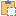#### GetProjectPosition Method

Gets the coordinates of a point in the ProjectLocation's coordinate system.

Namespace: Autodesk.Revit.DB
Assembly: RevitAPI (in RevitAPI.dll) Version: 20.0.0.0 (20.1.1.1)

# Syntax

C#
``````public ProjectPosition GetProjectPosition(
XYZ point
)``````
Visual Basic
``````Public Function GetProjectPosition ( _
point As XYZ _
) As ProjectPosition``````
Visual C++
``````public:
ProjectPosition^ GetProjectPosition(
XYZ^ point
)``````

#### Parameters

point
Type: Autodesk.Revit.DB XYZ

# Remarks

When getting this value, the North/South, East/West, and Elevation values report the coordinates of the point similar to the Revit command "Report Shared Coordinates". To get the values of the transformations applied by this project location, pass XYZ.Zero.

If the project has acquired shared coordinates from a linked model, the shared coordinate transformation will be reflected in the coordinates of the transformed point returned by this property.

# ExamplesCopy C#
``````//get and set the value of the projectlocation
public ProjectLocation GetProjectLocation(Autodesk.Revit.DB.Document document)
{
ProjectLocation currentLocation = document.ActiveProjectLocation;

//get the project position
XYZ origin = new XYZ(0, 0, 0);

const double angleRatio = Math.PI / 180;   // angle conversion factor

ProjectPosition projectPosition = currentLocation.GetProjectPosition(origin);
//Angle from True North
double angle = 30.0 * angleRatio;   // convert degrees to radian
double eastWest = 30.0;     //East to West offset
double northSouth = 23.32;   //North to South offset
double elevation = 22.14;    //Elevation above ground level

//create a new project position
ProjectPosition newPosition =
document.Application.Create.NewProjectPosition(eastWest, northSouth, elevation, angle);

if (null != newPosition)
{
//set the value of the project position
currentLocation.SetProjectPosition(origin, newPosition);
}

return currentLocation;
}``````Copy VB.NET
``````'get and set the value of the projectlocation
Public Function GetProjectLocation(document As Autodesk.Revit.DB.Document) As ProjectLocation
Dim currentLocation As ProjectLocation = document.ActiveProjectLocation

'get the project position
Dim origin As New XYZ(0, 0, 0)

Const angleRatio As Double = Math.PI / 180
' angle conversion factor
Dim projectPosition As ProjectPosition = currentLocation.GetProjectPosition(origin)
'Angle from True North
Dim angle As Double = 30.0 * angleRatio
Dim eastWest As Double = 30.0
'East to West offset
Dim northSouth As Double = 23.32
'North to South offset
Dim elevation As Double = 22.14
'Elevation above ground level
'create a new project position
Dim newPosition As ProjectPosition = document.Application.Create.NewProjectPosition(eastWest, northSouth, elevation, angle)

If newPosition IsNot Nothing Then
'set the value of the project position
currentLocation.SetProjectPosition(origin, newPosition)
End If

Return currentLocation
End Function``````

# Exceptions

Exception Condition
Autodesk.Revit.Exceptions ArgumentNullException A non-optional argument was NULL
Autodesk.Revit.Exceptions InvalidOperationException Unable to use the project position's transform to calculate the point.